1. /
2. CBSE
3. /
4. Class 07
5. /
6. Mathematics
7. /
8. NCERT Solutions for Class...

# NCERT Solutions for Class 7 Maths Exercise 6.5### myCBSEguide App

Download the app to get CBSE Sample Papers 2023-24, NCERT Solutions (Revised), Most Important Questions, Previous Year Question Bank, Mock Tests, and Detailed Notes.

NCERT solutions for Maths Triangles and its Properties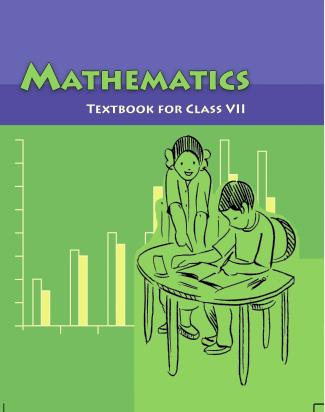## NCERT Solutions for Class 7 Maths Triangles and its Properties

###### Question 1.PQR is a triangle, right angled at P. If PQ = 10 cm and PR = 24 cm, find QR.

Given: PQ = 10 cm, PR = 24 cm

Let QR be {tex}x{/tex} cm.

In right angled triangle QPR,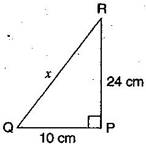{tex}{\left( {Hypotenuse} \right)^2} = {\text{ }}{\left( {Base} \right)^2} + {\text{ }}{\left( {Perpendicular} \right)^2}{/tex}

[By Pythagoras theorem]

{tex} \Rightarrow {/tex} {tex}{\left( {QR} \right)^2} = {\text{ }}{\left( {PQ} \right)^2} + {\text{ }}{\left( {PR} \right)^2}{/tex}

{tex} \Rightarrow {/tex} {tex}{x^2} = {\left( {10} \right)^2} + {\left( {24} \right)^2}{/tex}

{tex} \Rightarrow {/tex} {tex}{x^2}{/tex} = 100 + 576 = 676

{tex} \Rightarrow {/tex} {tex}x = \sqrt {676} {/tex} = 26 cm

Thus, the length of QR is 26 cm.

NCERT Solutions for Class 7 Maths Exercise 6.5

###### Question 2.ABC is a triangle, right angled at C. If AB = 25 cm and AC = 7 cm, find BC.

Given: AB = 25 cm, AC = 7 cm

Let BC be {tex}x{/tex} cm.

In right angled triangle ACB,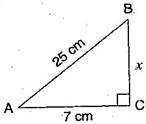{tex}{\left( {Hypotenuse} \right)^2} = {\text{ }}{\left( {Base} \right)^2} + {\text{ }}{\left( {Perpendicular} \right)^2}{/tex}

[By Pythagoras theorem]

{tex} \Rightarrow {/tex} {tex}{\left( {AB} \right)^2} = {\text{ }}{\left( {AC} \right)^2} + {\text{ }}{\left( {BC} \right)^2}{/tex}

{tex} \Rightarrow {/tex} {tex}{\left( {25} \right)^2} = {\left( 7 \right)^2} + {x^2}{/tex}

{tex} \Rightarrow {/tex} 625 = 49 + {tex}{x^2}{/tex}

{tex} \Rightarrow {/tex} {tex}{x^2}{/tex} = 625 – 49 = 576

{tex} \Rightarrow {/tex} {tex}x = \sqrt {576} {/tex} = 24 cm

Thus, the length of BC is 24 cm.

NCERT Solutions for Class 7 Maths Exercise 6.5

###### Question 3.A 15 m long ladder reached a window 12 m high from the ground on placing it against a wall at a distance {tex}a.{/tex} Find the distance of the foot of the ladder from the wall.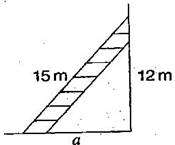Let AC be the ladder and A be the window.

Given: AC = 15 m, AB = 12 m, CB = {tex}a{/tex} m

In right angled triangle ACB,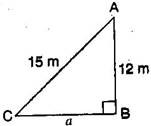{tex}{\left( {Hypotenuse} \right)^2} = {\text{ }}{\left( {Base} \right)^2} + {\text{ }}{\left( {Perpendicular} \right)^2}{/tex}

[By Pythagoras theorem]

{tex} \Rightarrow {/tex} {tex}{\left( {AC} \right)^2} = {\text{ }}{\left( {CB} \right)^2} + {\text{ }}{\left( {AB} \right)^2}{/tex}

{tex} \Rightarrow {/tex} {tex}{\left( {15} \right)^2} = {\left( a \right)^2} + {\left( {12} \right)^2}{/tex}

{tex} \Rightarrow {/tex} 225 = {tex}{a^2}{/tex} + 144

{tex} \Rightarrow {/tex} {tex}{a^2}{/tex} = 225 – 144 = 81

{tex} \Rightarrow {/tex} {tex}a = \sqrt {81} {/tex} = 9 cm

Thus, the distance of the foot of the ladder from the wall is 9 m.

NCERT Solutions for Class 7 Maths Exercise 6.5

###### Question 4.Which of the following can be the sides of a right triangle?
1. 2.5 cm, 6.5 cm, 6 cm
2. 2 cm, 2 cm, 5 cm
3. 1.5 cm, 2 cm, 2.5 cm

In the case of right angled triangles, identify the right angles.

Let us consider, the larger side be the hypotenuse and also using Pythagoras theorem,

{tex}{\left( {Hypotenuse} \right)^2} = {\text{ }}{\left( {Base} \right)^2} + {\text{ }}{\left( {Perpendicular} \right)^2}{/tex}

(i) 2.5 cm, 6.5 cm, 6 cm

In {tex}\Delta {\text{ABC}},{/tex} {tex}{\left( {{\text{AC}}} \right)^2} = {\left( {{\text{AB}}} \right)^2} + {\left( {{\text{BC}}} \right)^2}{/tex}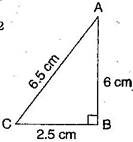L.H.S. = {tex}{\left( {6.5} \right)^2}{/tex} = 42.25 cm

R.H.S. = {tex}{\left( 6 \right)^2} + {\left( {2.5} \right)^2}{/tex} = 36 + 6.25 = 42.25 cm

Since, L.H.S. = R.H.S.

Therefore, the given sides are of the right angled triangle.

Right angle lies on the opposite to the greater side 6.5 cm, i.e., at B.

(ii) 2 cm, 2 cm, 5 cm

{tex}{\left( 5 \right)^2} = {\left( 2 \right)^2} + {\left( 2 \right)^2}{/tex}

L.H.S. = {tex}{\left( 5 \right)^2}{/tex} = 25

R.H.S. = {tex}{\left( 2 \right)^2} + {\left( 2 \right)^2}{/tex} = 4 + 4 = 8

Since, L.H.S. {tex} \ne {/tex} R.H.S.

Therefore, the given sides are not of the right angled triangle.

(iii) 1.5 cm, 2 cm, 2.5 cm

In {tex}\Delta {/tex}PQR, {tex}{\left( {{\text{PR}}} \right)^2} = {\left( {{\text{PQ}}} \right)^2} + {\left( {{\text{RQ}}} \right)^2}{/tex}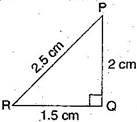L.H.S. = {tex}{\left( {2.5} \right)^2}{/tex} = 6.25 cm

R.H.S. = {tex}{\left( {1.5} \right)^2} + {\left( 2 \right)^2}{/tex} = 2.25 + 4 = 6.25 cm

Since, L.H.S. = R.H.S.

Therefore, the given sides are of the right angled triangle.

Right angle lies on the opposite to the greater side 2.5 cm, i.e., at Q.

NCERT Solutions for Class 7 Maths Exercise 6.5

###### Question 5.A tree is broken at a height of 5 m from the ground and its top touches the ground at a distance of 12 m from the base of the tree. Find the original height of the tree.

Let A’CB represents the tree before it broken at the point C and let the top A’ touches the ground at A after it broke. Then {tex}\Delta {\text{ABC}}{/tex} is a right angled triangle, right angled at B.

AB = 12 m and BC = 5 m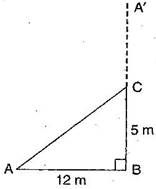Using Pythagoras theorem, In {tex}\Delta {\text{ABC}}{/tex}

{tex}{\left( {{\text{AC}}} \right)^2} = {\left( {{\text{AB}}} \right)^2} + {\left( {{\text{BC}}} \right)^2}{/tex}

{tex} \Rightarrow {/tex} {tex}{\left( {{\text{AC}}} \right)^2} = {\left( {12} \right)^2} + {\left( 5 \right)^2}{/tex}

{tex} \Rightarrow {/tex} {tex}{\left( {{\text{AC}}} \right)^2} = 144 + 25{/tex}

{tex} \Rightarrow {/tex} {tex}{\left( {{\text{AC}}} \right)^2} = 169{/tex}

{tex} \Rightarrow {/tex} AC = 13 m

Hence, the total height of the tree = AC + CB = 13 + 5 = 18 m.

NCERT Solutions for Class 7 Maths Exercise 6.5

###### Question 6.Angles Q and R of a {tex}\Delta {/tex}PQR are {tex}25^\circ {/tex} and {tex}65^\circ .{/tex}

Write which of the following is true:

1. {tex}P{Q^2} + {\text{ }}Q{R^2} = {\text{ }}R{P^2}{/tex}
2. {tex}P{Q^2} + {\text{ }}R{P^2} = {\text{ }}Q{R^2}{/tex}
3. {tex}R{P^2} + {\text{ }}Q{R^2} = {\text{ }}P{Q^2}{/tex}

{tex}25^\circ {/tex} {tex}65^\circ {/tex}

In {tex}\Delta {/tex}PQR,

{tex}\angle {/tex}PQR + {tex}\angle {/tex}QRP + {tex}\angle {/tex}RPQ = {tex}180^\circ {/tex}

[By Angle sum property of a {tex}\Delta {/tex} ]

{tex} \Rightarrow {/tex} {tex}25^\circ + 65^\circ + \angle {\text{RPQ = 180}}^\circ {/tex}

{tex} \Rightarrow {/tex} {tex}90^\circ + \angle {\text{RPQ = 180}}^\circ {/tex}

{tex} \Rightarrow {/tex} {tex}\angle {/tex}RPQ = {tex}180^\circ – 90^\circ = 90^\circ {/tex}

Thus, {tex}\Delta {/tex}PQR is a right angled triangle, right angled at P.

{tex}\therefore {/tex} (Hypotenuse)2 = (Base)2 + (Perpendicular)2 [By Pythagoras theorem]

{tex} \Rightarrow {/tex} {tex}{\left( {{\text{QR}}} \right)^2} = {\left( {{\text{PR}}} \right)^2} + {\left( {{\text{QP}}} \right)^2}{/tex}

Hence, Option (ii) is correct.

NCERT Solutions for Class 7 Maths Exercise 6.5

###### Question 7.Find the perimeter of the rectangle whose length is 40 cm and a diagonal is 41 cm.

Given diagonal (PR) = 41 cm, length (PQ) = 40 cm

Let breadth (QR) be {tex}x{/tex} cm.

Now, in right angled triangle PQR,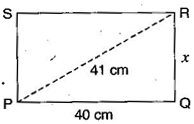{tex}{\left( {{\text{PR}}} \right)^2} = {\left( {{\text{RQ}}} \right)^2} + {\left( {{\text{PQ}}} \right)^2}{/tex}

[By Pythagoras theorem]

{tex} \Rightarrow {/tex} {tex}{\left( {41} \right)^2} = {x^2} + {\left( {40} \right)^2}{/tex}

{tex} \Rightarrow {/tex} 1681 = {tex}{x^2}{/tex} + 1600 {tex} \Rightarrow {/tex} {tex}{x^2}{/tex} = 1681 – 1600

{tex} \Rightarrow {/tex} {tex}{x^2}{/tex} = 81 {tex} \Rightarrow {/tex} {tex}x = \sqrt {81} = 9{/tex} cm

Therefore the breadth of the rectangle is 9 cm.

Perimeter of rectangle = 2(length + breadth)

= 2 (9 + 49)

= 2 x 49 = 98 cm

Hence the perimeter of the rectangle is 98 cm.

NCERT Solutions for Class 7 Maths Exercise 6.5

###### Question 8.The diagonals of a rhombus measure 16 cm and 30 cm. Find its perimeter.

Given: Diagonals AC = 30 cm and DB = 16 cm.

Since the diagonals of the rhombus bisect at right angle to each other.

Therefore, OD = {tex}\frac{{{\text{DB}}}}{2} = \frac{{16}}{2}{/tex} = 8 cm

And OC = {tex}\frac{{{\text{AC}}}}{2} = \frac{{30}}{2}{/tex} = 15 cm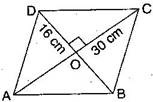Now, In right angle triangle DOC,

{tex}{\left( {{\text{DC}}} \right)^2} = {\left( {{\text{OD}}} \right)^2} + {\left( {{\text{OC}}} \right)^2}{/tex} [By Pythagoras theorem]

{tex} \Rightarrow {/tex} {tex}{\left( {{\text{DC}}} \right)^2} = {\left( 8 \right)^2} + {\left( {15} \right)^2}{/tex}

{tex} \Rightarrow {/tex} {tex}{\left( {{\text{DC}}} \right)^2}{/tex} = 64 + 225 = 289

{tex} \Rightarrow {/tex} DC = {tex}\sqrt {289} {/tex} = 17 cm

Perimeter of rhombus = 4 x side

= 4 x 17 = 68 cm

Thus, the perimeter of rhombus is 68 cm.

## NCERT Solutions for Class 7 Maths Exercise 6.5

NCERT Solutions Class 7 Maths PDF (Download) Free from myCBSEguide app and myCBSEguide website. Ncert solution class 7 Maths includes text book solutions from Class 7 Maths Book . NCERT Solutions for CBSE Class 7 Maths have total 15 chapters. 7 Maths NCERT Solutions in PDF for free Download on our website. Ncert Maths class 7 solutions PDF and Maths ncert class 7 PDF solutions with latest modifications and as per the latest CBSE syllabus are only available in myCBSEguide.

## CBSE app for Students

To download NCERT Solutions for Class 7 Maths, Social Science Computer Science, Home Science, Hindi English, Maths Science do check myCBSEguide app or website. myCBSEguide provides sample papers with solution, test papers for chapter-wise practice, NCERT solutions, NCERT Exemplar solutions, quick revision notes for ready reference, CBSE guess papers and CBSE important question papers. Sample Paper all are made available through the best app for CBSE students and myCBSEguide website.Test Generator

Create question paper PDF and online tests with your own name & logo in minutes.myCBSEguide

Question Bank, Mock Tests, Exam Papers, NCERT Solutions, Sample Papers, Notes

### 18 thoughts on “NCERT Solutions for Class 7 Maths Exercise 6.5”

1. Thanks

2. Thanks

3. Thanks anamika tyagi

4. thanks

5. In a 7 question you write 2(9+49) but it is 2(9+40)

6. In question 7 how it come 49cm

7. Very good

8. Thanks????????????????????????????????????

9. Thanks????????????????????????????????????

10. 7th qns confused me
But it is ok ?

11. THANK YOU

12. Very useful and we can understand very easily.